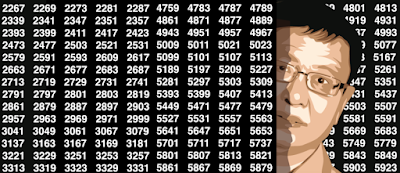## Wednesday, March 13, 2019

### How to Automatically Determine the Number of Clusters in your Data - and more

Determining the number of clusters when performing unsupervised clustering is a tricky problem. Many data sets don't exhibit well separated clusters, and two human beings asked to visually tell the number of clusters by looking at a chart, are likely to provide two different answers. Sometimes clusters overlap with each other, and large clusters contain sub-clusters, making a decision not easy.
For instance, how many clusters do you see in the picture below? What is the optimum number of clusters? No one can tell with certainty, not AI, not a human being, not an algorithm.
How many clusters here? (source: see here)
In the above picture, the underlying data suggests that there are three main clusters. But an answer such as 6 or 7, seems equally valid.
A number of empirical approaches have been used to determine the number of clusters in a data set. They usually fit into two categories:
• Model fitting techniques: an example is using a mixture model to fit with your data, and determine the optimum number of components; or use density estimation techniques, and test for the number of modes (see here.) Sometimes, the fit is compared with that of a model where observations are uniformly distributed on the entire support domain, thus with no cluster; you may have to estimate the support domain in question, and assume that it is not  made of disjoint sub-domains; in many cases, the convex hull of your data set, as an estimate of the support domain, is good enough.
• Visual techniques: for instance, the silhouette or elbow rule (very popular.)
In both cases, you need a criterion to determine the optimum number of clusters. In the case of the elbow rule, one typically uses the percentage of unexplained variance. This number is 100% with zero cluster, and it decreases (initially sharply, then more modestly) as you increase the number of clusters in your model. When each point constitutes a cluster, this number drops to 0.  Somewhere in between, the curve that displays your criterion, exhibits an elbow (see picture below), and that elbow determines the number of clusters. For instance, in the chart below, the optimum number of clusters is 4.
The elbow rule tells you that here, your data set has 4 clusters (elbow strength in red)
Good references on the topic are available. Some R functions are available too, for instance fviz_nbclust. However, I could not find in the literature, how the elbow point is explicitly computed. Most references mention that it is mostly hand-picked by visual inspection, or based on some predetermined but arbitrary threshold. In the next section, we solve this problem.